Nuclear Physics MCQ Level – 1

# Nuclear Physics MCQ Level – 1

Test Description

## 10 Questions MCQ Test Topic wise Tests for IIT JAM Physics | Nuclear Physics MCQ Level – 1

Nuclear Physics MCQ Level – 1 for IIT JAM 2023 is part of Topic wise Tests for IIT JAM Physics preparation. The Nuclear Physics MCQ Level – 1 questions and answers have been prepared according to the IIT JAM exam syllabus.The Nuclear Physics MCQ Level – 1 MCQs are made for IIT JAM 2023 Exam. Find important definitions, questions, notes, meanings, examples, exercises, MCQs and online tests for Nuclear Physics MCQ Level – 1 below.
Solutions of Nuclear Physics MCQ Level – 1 questions in English are available as part of our Topic wise Tests for IIT JAM Physics for IIT JAM & Nuclear Physics MCQ Level – 1 solutions in Hindi for Topic wise Tests for IIT JAM Physics course. Download more important topics, notes, lectures and mock test series for IIT JAM Exam by signing up for free. Attempt Nuclear Physics MCQ Level – 1 | 10 questions in 30 minutes | Mock test for IIT JAM preparation | Free important questions MCQ to study Topic wise Tests for IIT JAM Physics for IIT JAM Exam | Download free PDF with solutions
 1 Crore+ students have signed up on EduRev. Have you?
Nuclear Physics MCQ Level – 1 - Question 1

### Order of ionization power of α,β, and γ rays is

Detailed Solution for Nuclear Physics MCQ Level – 1 - Question 1

Since α rays has more charge than β and γ has no charge therefore order of ionization is α > β > γ.

The correct answer is: α > β > γ

Nuclear Physics MCQ Level – 1 - Question 2

### The binding energies of the nuclei Pn and  Q2n are x and y  joules. If 2x > y  then the energy released in the reaction  Pn + Pn → Q2n,  will be

Detailed Solution for Nuclear Physics MCQ Level – 1 - Question 2

The correct answer is: -(2x - y)

Nuclear Physics MCQ Level – 1 - Question 3

### A radioactive element Th(84Po216) can undergo α and β are type of disintegration with half lives, T1 and T2 respectively. Then the half life of Th is .

Detailed Solution for Nuclear Physics MCQ Level – 1 - Question 3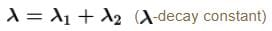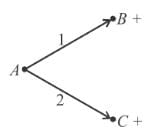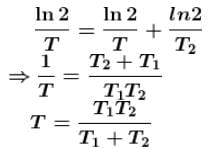The correct answer is: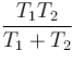Nuclear Physics MCQ Level – 1 - Question 4

The order of magnitude of the density of nuclear matter is :

Detailed Solution for Nuclear Physics MCQ Level – 1 - Question 4

The correct answer is: 1017 kg/m

Nuclear Physics MCQ Level – 1 - Question 5

The value of binding energy for 1H22He428Fe5692U235  are 2.22, 28.3, 492 and 1786 units respectively. The most stable nucleus is :

Detailed Solution for Nuclear Physics MCQ Level – 1 - Question 5

Nuclear Physics MCQ Level – 1 - Question 6

The specific activity of radium is nearly

Detailed Solution for Nuclear Physics MCQ Level – 1 - Question 6

Nuclear Physics MCQ Level – 1 - Question 7

In equation 92U235 + 0n→ 56Ba144 + 36Kr89 + X ; X is :

Detailed Solution for Nuclear Physics MCQ Level – 1 - Question 7

The correct answer is: 3 0n1

Nuclear fission equation

92U235 +  0n→ 56Ba144 + 36Kr89 + 3 0n
Hence particle x is neutron.

Nuclear Physics MCQ Level – 1 - Question 8

Masses of nucleus, neutron an protons are M, mn and mrespectively. If nucleus has been divided into neutrons and protons, then

Detailed Solution for Nuclear Physics MCQ Level – 1 - Question 8

The correct answer is: M < (A - Z)mn + Zmp

Nuclear Physics MCQ Level – 1 - Question 9

In most stable nuclei neutron number N and proton number Z has the relation

Detailed Solution for Nuclear Physics MCQ Level – 1 - Question 9

The correct answer is: N ≥ Z

Nuclear Physics MCQ Level – 1 - Question 10

The graph between the binding energy per nucleon (E) and atomic mass number (A) is as :

Detailed Solution for Nuclear Physics MCQ Level – 1 - Question 10

The correct answer is: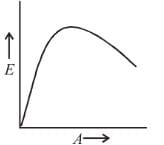## Topic wise Tests for IIT JAM Physics

217 tests
 Use Code STAYHOME200 and get INR 200 additional OFF Use Coupon Code
Information about Nuclear Physics MCQ Level – 1 Page
In this test you can find the Exam questions for Nuclear Physics MCQ Level – 1 solved & explained in the simplest way possible. Besides giving Questions and answers for Nuclear Physics MCQ Level – 1, EduRev gives you an ample number of Online tests for practice

217 tests